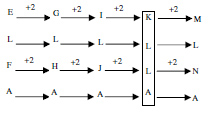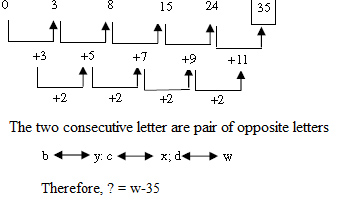# Miracle Soft Reasoning Question

DIRECTIONS for questions 1-2: Solve the following question and mark the most appropriate option.
1. The set which resembles the given set 1, 5, 12 is
1. 7, 11,35
2. 4, 8, 24
3. 10, 14, 44
4. 9, 13, 42
1 + 5 = 6 and 6 × 2 = 12 Similarly, 4 + 8 = 12 and 12 × 2 = 24 Hence, the answer is 4, 8, 24
2. ELFA, GLHA, ILJA, ? , MLNA
1. OLPA
2. KLMA
3. LLMA
4. KLLADIRECTIONS for the question 3 to 4: What should come in place of question mark (?) in the following number/alphabetic series?

# Complete Test Series for Wipro Bag your Dream Job Today Comprehensive Online Tests Inclusive Prep for All Placement Exams Acquire Essential Domain Skills

1. 4, 10, 24, 54, ?, 242
1. 80
2. 116
3. 110
4. 114
4 x 2 + 2 = 10
10 x 2 + 4 = 24
24 x 2 + 6 = 54
54 x 2 + 8 = 116
116 x 2 + 10 = 242
So 116 should be the answer.
2. F G H J K L N O P R S ?
1. T
2. M
3. Q
4. N
FGH are three consecutive alphabets. Then 1 alphabet I is skipped and next three alphabets JKL are written and so on.
Continuing this way the group RS should have T as the missing alphabet.
So option A.
DIRECTIONS for questions 5-8: Solve the following question and mark the best possible option.
3. b-0, y-3, c-8, x-15, d-24,?
1. e-48
2. w-35
3. w-39
4. v-304. The average age of father and his son is 22 years. The ratio of their ages is 10 : 1 respec-tively. What is the age of the son??
1. 24
2. 4
3. 40
4. 14
Suppose the age of son is x years
Therefore, age of father = 10x years
According to question
(10x + x)/2 = 22
11x = 44
x = 4 years
Hence, age of son is 4 years
5. Veni is an year older than Smith. Smith is two years older than Salim. Raju is an year older than Salim. Who is the youngest of all ?
1. Raju
2. Salim
3. Veni
4. Smith
Suppose the age of Salim is x years
Age of Raju = x + 1 year
Age of Smith = x + 2 years
Age of veni = x + 3 years
Therefore, Salim is the youngest of all.
6. Rachel starts walking towards North. After walking 15 meteres, she turns towards South and walks 20 metres. She then turns towards East and walks 10 meters. Then, again, she walks 5 metres towards North. How far is she from her starting point and in which direction?
1. 10 metres, West
2. 5 metres, East
3. 5 metres, North
4. 10 metres, EastRequired distance = AE = 10m
Rachel is 10 metres towards East from the starting point
DIRECTIONS for questions 9-10: In the following question, one word is followed by four/five different words in options, check and mark that word as your answer which cannot be made by using the letters of the key word given in the question.
7. HETEROGENOUS
1. REGENT
2. GENERATE
3. STORE
4. ROUTE